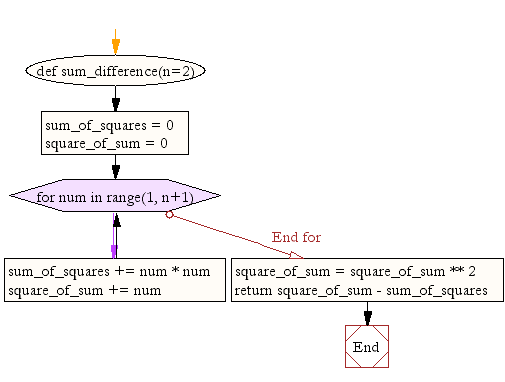﻿ Python Math: Calculate the difference between the squared sum and the sum of the squared of first n natural numbers - w3resource# Python Math: Calculate the difference between the squared sum and the sum of squared of first n natural numbers

## Python Math: Exercise-11 with Solution

Write a Python program to calculate the difference between the squared sum of first n natural numbers and the sum of squared first n natural numbers.(default value of number=2).

Sample Solution:-

Python Code:

``````def sum_difference(n=2):
sum_of_squares = 0
square_of_sum = 0
for num in range(1, n+1):
sum_of_squares += num * num
square_of_sum += num

square_of_sum = square_of_sum ** 2

return square_of_sum - sum_of_squares

print(sum_difference(12))
```
```

Sample Output:

```5434
```

Pictorial Presentation:Flowchart:## Visualize Python code execution:

The following tool visualize what the computer is doing step-by-step as it executes the said program:

Python Code Editor:

Have another way to solve this solution? Contribute your code (and comments) through Disqus.

What is the difficulty level of this exercise?

Test your Programming skills with w3resource's quiz.

﻿

```>>> students = [{'name': 'John', 'score': 98}, {'name': 'Mike', 'score': 94}, {'name': 'Jennifer', 'score': 99}]